## SOCNET@LISTS.UFL.EDU

#### View:

 Message:[First|Previous|Next|Last] By Topic:[First|Previous|Next|Last] By Author:[First|Previous|Next|Last] Font: Proportional Font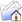LISTSERV Archives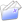SOCNET HomeSOCNET November 2009

Subject:Re: A computational problem with triangular grids

From:Date:Fri, 6 Nov 2009 16:48:34 +0200

Content-Type:text/plain

Parts/Attachments: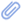text/plain (72 lines)
 ```***** To join INSNA, visit http://www.insna.org ***** Thanks to Mark Newman and Christian Pich for providing the following answer to my question: The simplest representation uses a square grid, with additional diagonal edges one way across each square. Thus the grid element (i,j) is a neighbor of:  (i+1,j)  (i-1,j)  (i,j+1)  (i,j-1)  (i+1,j+1)  (i-1,j-1) In this way, enumerating vertices in row-major order (i.e., all of the first lattice row, then all of the second row, and so forth), the adjacency matrix of a triangular grid of n^2 elements (without periodical suturing) is a matrix of order n^2, containing n^2 blocks each-one of order n. Among all these blocks, the nonzero blocks are the following: (i) n diagonal blocks with 1s only just above and below the diagonal. (ii) n - 1 blocks just above the diagonal blocks, which are starting from the block position (1,2) and going up to the block position (n-1,n) and each one includes 1s only on its diagonal and just above the diagonal. (iii) n - 1 blocks just below the diagonal blocks, which are starting from the block position (2,1) and going up to the block position (n,n-1) and each one includes 1s only on its diagonal and just below the diagonal. Best, --Moses On Thu, Nov 5, 2009 at 6:19 PM, Moses Boudourides <[log in to unmask]> wrote: > Hello, > > Say we have a triangular grid (tiling) formed by nxm vertices (i.e., a > graph in which all internal vertices have degree 6). What I want to > obtain is the structure of the matrix (or list) representation of its > edges. Of course, this depends on the enumeration of vertices. Thus, > the question is what re-ordering gives the simplest general form of > such a matrix (or list) and what is exactly this form? > > My motivation comes from that I want to make a simulation of a network > influence situation in which edges (ties) are influenced by the > incident ones (than vertices being influenced by the neighboring ones) > according to certain rules. In fact, first I'm assuming that the grid > is signed and that signs of edges may change in such a way that they > follow the structural stability or instability of the triangles with > common edges. > > I would appreciate any help or hints on how I might proceed in dealing > with this problem. > > Best, > > --Moses > _____________________________________________________________________ SOCNET is a service of INSNA, the professional association for social network researchers (http://www.insna.org). To unsubscribe, send an email message to [log in to unmask] containing the line UNSUBSCRIBE SOCNET in the body of the message.```

#### Search Archives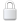Log In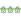Get Password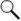Search Archives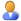Subscribe or Unsubscribe